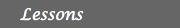MATH EXPLORERS' CLUB Cornell Department of Mathematics

# Ehrenfeucht-Fraïssé games

 Introduction1. History of EF games 2. Description 3. Examples 4. FO logic for graphs 5. EF games & FO logic 6. Applications References## History of the Ehrenfeucht-Fraïssé games

In 1930 Alfred Tarski formulated the notion of two structures A and B being elementarily equivalent, meaning that exactly the same first-order sentences are true in A as are true in B. At a conference in Princeton in 1946 he described this notion and expressed the hope that it would be possible to develop a theory of it that would be "as deep as the notions of isomorphism, now in use".

One natural part of such a theory would be a purely structural necessary and sufficient condition for two structures to be elementarily equivalent. Roland Fraïssé, a French-Algerian, was the first to find a usable necessary and sufficient condition. It was rediscovered a few years later by the Kazakh logician A. D. Taimanov, and it was reformulated in terms of games by the Polish logician Andrzej Ehrenfeucht. The games are now known as Ehrenfeucht-Fraïssé games, or sometimes as back-and-forth games. They have turned out to be one of the most versatile ideas in twentieth-century logic. They adapt fruitfully to a wide range of logics and structures.

The notion of 'structures' is very general and quite vague. The structures that these lessons deal with will be graphs. In a back-and-forth game there are two structures A and B, and two players who are commonly called Spoiler and Duplicator. (Sometimes using the same initials, they are also called Samson and Delilah; this places Spoiler as the male player, and Duplicator as the female player).

Each round in the game consists of a move of Spoiler, followed by a move of Duplicator. Spoiler chooses an element of one of the two graphs, and Duplicator must then choose an element of the other graph. So after n rounds, two sequences have been chosen, one from A and one from B: (a1, a2, … , an; b1, b2, … , bn). This position is a win for Spoiler if and only if some atomic formula is satisfied by a1, a2, … , an in A but not by b1, b2, … , bn in B, or vice versa. For instance, if, after 4 steps, a1, a2, a3, a4 is a subgraph of A with the property that there is an edge between a4 and each of the other three nodes a1, a2 and a3, and b1, b2, b3, b4 is a subgraph of B where b4 is not connected to each of the other four nodes b1, b2 and b3 , then the Spoiler wins the 4 rounds EF game on the graphs A and B.

The condition for Duplicator to win is different in different forms of the game. If the number of rounds is known in advance, say n = 4, a play (a1, a2, … , a4; b1, b2, … , b4) is a win for the Duplicator if the Spoiler has not won rounds 1, 2, 3 and 4. If we play an infinite number of rounds, a play (a1, a2, … ; b1, b2, … ) is a win for Duplicator if and only if no initial part of it is a win for Spoiler (i.e. she wins if she hasn't lost by any finite stage).

The two structures A and B are said to be back-and-forth equivalent if Duplicator has a winning strategy for EF(A, B)(EF game with infinite number of rounds), and n-equivalent if she has a winning strategy for EFn(A, B)(EF game on n rounds). One can prove that if A and B are n-equivalent for every natural number n, then they are elementarily equivalent. This means that there is no first order sentence which is true on A and false on B. On the other hand a winning strategy for Spoiler in EFn(A, B) can be converted into a first-order sentence that is true in exactly one of A and B, and in which the nesting of quantifier scopes has at most n levels. So we have our necessary and sufficient condition for elementary equivalence, and a bit more besides.

The theory behind back-and-forth games uses very few assumptions about the logic in question. As a result, these games are one of the few model-theoretic techniques that apply as well to finite structures as they do to infinite ones, and this makes them one of the cornerstones of theoretical computer science. One can use them to measure the expressive strength of formal languages, for example database query languages. A typical result might say, for example, that a certain language can not distinguish between "even" and "odd"; we would prove this by finding, for each level n of complexity of formulas of the language, a pair of finite structures for which Duplicator has a winning strategy in the back-and-forth game of level n, but one of the structures has an even number of elements and the other has an odd number.

 Top Next lesson